Do you like the article?
Share it with others —
Use new possibilities of MetaTrader 5

#### Similar articles# Creating an Indicator with Multiple Indicator Buffers for Newbies

MetaTrader 5Examples | 8 December 2010, 10:5323 4111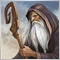### Introduction

In my previous articles "Custom Indicators in MQL5 for Newbies" and "Practical Implementation of Digital Filers in MQL5 for Beginners" I focused in details on structure of the indicator with one indicator buffer.

Obviously, such a method can be widely applied for writing custom indicators, but the real life can hardly be limited to their use, and thus it is time to approach more complex methods of building the indicator code. Fortunately, the capabilities of MQL5 are truly inexhaustible and are limited only by the RAM of our PCs.

### The Aroon Indicator as example of code doubling

The formula of this indicator contains two components: the bullish and the bearish indicators, which are plotted in a separate chart window:

BULLS =  (1 - (bar - SHIFT(MAX(HIGH(), AroonPeriod)))/AroonPeriod) * 100
BEARS = (1 - (bar - SHIFT(MIN (LOW (), AroonPeriod)))/AroonPeriod) * 100

where:

• BULLS - the Bull's strength;
• BEARS - the Bear's strength;
• SHIFT() - function of determining the index position of the bar;
• MAX() - function of searching for the maximum over the AroonPeriod period;
• MIN() - function of searching for the minimum over the AroonPeriod period;
• HIGH() and LOW() - the relevant price arrays;

Right from the formulas of the indicator, we can conclude that to construct an indicator, we must have only two indicator buffers, the indicator structure will differ very little from the structure of the SMA_1.mq5, considered in the previous article.

Practically, it is simply the same duplicated code, with different numbers of indicator buffers. So let's open the code of this indicator in the MetaEditor, and save as Aroon.mq5. Now, in the first 11 lines of the code, which relates to the copyright and its version number, we will replace only the name of the indicator:

```//+------------------------------------------------------------------+
//|                                                        Aroon.mq5 |
//|                        Copyright 2010, MetaQuotes Software Corp. |
//|                                              http://www.mql5.com |
//+------------------------------------------------------------------+
#property copyright "2010, MetaQuotes Software Corp."
//---- link to the author's site
//---- version number
#property version   "1.00"```

Next, in the 12th line of code, we need to change the plotting of the indicator from the basic chart window to the separate window:

```//---- plot indicator in the separate window
#property indicator_separate_window
```

Since this indicator has a completely different range of values, its plotting will be made in a separate window.

After this, in the following 4 lines of the code (the general indicator properties), we change the number of the used indicator buffers to two:

```//---- two buffers are used
#property indicator_buffers 2
//---- two plots are used
#property indicator_plots   2```

The next 10 lines of code are related to the plotting of the indicator from a specific indicator buffer, it's label must be duplicated, after which, we must replace all the indexes from 1 to 2. We must also change all of the labels of the indicator buffers:

```//+----------------------------------------------+
//| bullish strength indicator parameters        |
//+----------------------------------------------+
//---- drawing style = line
#property indicator_type1   DRAW_LINE
//---- drawing color = Lime
#property indicator_color1  Lime
//---- line style = solid line
#property indicator_style1  STYLE_SOLID
//---- line width = 1
#property indicator_width1  1
//---- label of the BullsAroon indicator
#property indicator_label1  "BullsAroon"
//+----------------------------------------------+
//|  bearish strength indicator parameters       |
//+----------------------------------------------+
//---- drawing style = line
#property indicator_type2   DRAW_LINE
//---- drawing color = Red
#property indicator_color2  Red
//---- line style = solid line
#property indicator_style2  STYLE_SOLID
//---- line width = 1
#property indicator_width2  1
//---- label of the BearsAroon indicator
#property indicator_label2  "BearsAroon"```

This indicator uses three horizontal levels with the values of 30, 50 and 70.

In order to plot these levels, we need to add five more code lines to the code of the indicator.

```//+----------------------------------------------+
//| Horizontal levels                            |
//+----------------------------------------------+
#property indicator_level1 70.0
#property indicator_level2 50.0
#property indicator_level3 30.0
#property indicator_levelcolor Gray
#property indicator_levelstyle STYLE_DASHDOTDOT```

For the indicator input parameters, in comparison to the previous indicator, everything remains the same, aside for small changes in the titles:

```//+----------------------------------------------+
//| Indicator input parameters                   |
//+----------------------------------------------+
input int AroonPeriod = 9; // Period
input int AroonShift = 0;  // Horizontal shift of the indicator in bars ```

Now, however, there will be two arrays, which will be used as indicator buffers, and they will have appropriate names:

```//--- declare the dynamic arrays used further as indicator buffers
double BullsAroonBuffer[];
double BearsAroonBuffer[]; ```

We proceed in a completely same matter with the code of the OnInit() function.

First, we modify the lines of code of the zeroth buffer:

```//--- set BullsAroonBuffer dynamic array as indicator buffer
SetIndexBuffer(0, BullsAroonBuffer, INDICATOR_DATA);
//--- horizontal shift (AroonShift) of the indicator 1
PlotIndexSetInteger(0, PLOT_SHIFT, AroonShift);
//--- plot draw begin (AroonPeriod) of the indicator 1
PlotIndexSetInteger(0, PLOT_DRAW_BEGIN, AroonPeriod);
//--- label shown in DataWindow
PlotIndexSetString(0, PLOT_LABEL, "BearsAroon"); ```

After this, copy the entire code to the Windows clipboard, and paste it right after the same code.

Then, in the pasted code, we change the number of the indicator buffer from 0 to 1, change the name of the indicator array, and label of the indicator:

```//--- set BearsAroonBuffer dynamic array as indicator buffer
SetIndexBuffer(1, BearsAroonBuffer, INDICATOR_DATA);
//--- horizontal shift (AroonShift) of the indicator 2
PlotIndexSetInteger(1, PLOT_SHIFT, AroonShift);
//--- plot draw begin (AroonPeriod) of the indicator 2
PlotIndexSetInteger(1, PLOT_DRAW_BEGIN, AroonPeriod);
//--- label shown in DataWindow
PlotIndexSetString(1, PLOT_LABEL, "BullsAroon");  ```

The indicator short name has also undergone minor changes:

```//--- initialization of the variable for a short indicator name
string shortname;
StringConcatenate(shortname, "Aroon(", AroonPeriod, ", ", AroonShift, ")"); ```

Now, let's consider the accuracy of plotting the indicator. The actual range of the indicator is from 0 to 100, and this range is shown all the time.

In this situation, it is quite possible to use only the integer values of the indicator, plotted on the chart. For this reason, we use 0 for the numbers after the decimal point, for the indicator plotting:

```//--- set accuracy of drawing of indicator values
IndicatorSetInteger(INDICATOR_DIGITS, 0);```

In the SMA_1.mq5 indicator, we used the first form of the OnCalculate() function call.

It's is not suitable for the Aroon indicator, because of its lack of  the high[] and low[] price arrays. These arrays are available in the second calling form of this function. And, therefore, it is necessary to change the function's header:

```int OnCalculate(
const int rates_total,    // total bars on the current tick
const int prev_calculated,// total bars on the previous tick
const datetime& time[],
const double& open[],
const double& high[],     // price array of the maximum prices for the indicator calculations
const double& low[],      // price array of the minimum prices for the indicator calculations
const double& close[],
const long& tick_volume[],
const long& volume[],
)```

After this change, the use of the begin parameter, has lost all meaning, so it needs to be removed from the code!

The code for calculating the limits of variable changes of the operation cycle, the data verification for calculation sufficiency, has practically remained unchanged.

```//--- checking the number of bars
if (rates_total < AroonPeriod - 1) return(0);

//--- declare the local variables
int first, bar;
double BULLS, BEARS;

//--- calculation of the first (staring index) for the main loop
if (prev_calculated == 0)          // checking for the first call of the OnCalculate function
first = AroonPeriod - 1;       // starting index for calculating all of the bars
else first = prev_calculated - 1;  // starting index for calculating new bars```

However, the certain problems appear with the algorithms for calculating the indicator values. The problem is that MQL5 does not have the built-in functions to determine the indexes of the maximum and the minimum, for the period from the current bar, in the direction of the decreasing index.

One way out of this situation is to write these functions ourselves. Fortunately, such functions already exists in the ZigZag.mq5 indicator in the of custom indicators, located in the "MetaTrader5\MQL5\Indicators\Examples" folder.

The easiest way out - is to select the code of these functions in the ZigZag.mq5 indicator, copy them to the Windows clipboard, and paste them into our code, for example, right after the describing the OnInit() function, at the global level:

```//+------------------------------------------------------------------+
//|  searching index of the highest bar                              |
//+------------------------------------------------------------------+
int iHighest(const double &array[], // array for searching for the index of the maximum element
int count,            // number of the elements in the array (in the decreasing order),
int startPos          // starting index
)
{
//---+
int index = startPos;

//---- checking the starting index
if (startPos < 0)
{
Print("Incorrect value in the function iHighest, startPos = ", startPos);
return (0);
}
//---- checking the startPos values
if (startPos - count < 0) count = startPos;

double max = array[startPos];

//---- index search
for(int i = startPos; i > startPos - count; i--)
{
if(array[i] > max)
{
index = i;
max = array[i];
}
}
//---+ return of the index of the largest bar
return(index);
}
//+------------------------------------------------------------------+
//|  searching index of the lowest bar                               |
//+------------------------------------------------------------------+
int iLowest(
const double &array[], // array for searching for the index of the maximum element
int count,            // number of the elements in the array (in the decreasing order),
int startPos          // starting index
)
{
//---+
int index = startPos;

//--- checking the stating index
if (startPos < 0)
{
Print("Incorrect value in the iLowest function, startPos = ",startPos);
return(0);
}

//--- checking the startPos value
if (startPos - count < 0) count = startPos;

double min = array[startPos];

//--- index search
for(int i = startPos; i > startPos - count; i--)
{
if (array[i] < min)
{
index = i;
min = array[i];
}
}
//---+ return of the index of the smallest bar
return(index);
}```

After this, the code of the OnCalculate() function will look the following way:

```//+------------------------------------------------------------------+
//| Custom indicator iteration function                              |
//+------------------------------------------------------------------+
int OnCalculate( const int rates_total,    // total number of bars on the current tick
const int prev_calculated,// number of calculated bars on the previous tick
const datetime& time[],
const double& open[],
const double& high[],     // price array for the maximum price for the indicator calculation
const double& low[],      // price array for the minimum price for the indicator calculation
const double& close[],
const long& tick_volume[],
const long& volume[],
)
{
//---+
//--- checking the number of bars
if (rates_total < AroonPeriod - 1)
return(0);

//--- declare the local variables
int first, bar;
double BULLS, BEARS;

//--- calculation of the starting bar number
if (prev_calculated == 0)  // checking for the first start of the indicator calculation
first = AroonPeriod - 1; // starting number for the calculation of all of the bars

else first = prev_calculated - 1; // starting number for the calculation of new bars

//--- main loop
for(bar = first; bar < rates_total; bar++)
{
//--- calculation of values
BULLS = 100 - (bar - iHighest(high, AroonPeriod, bar) + 0.5) * 100 / AroonPeriod;
BEARS = 100 - (bar - iLowest (low,  AroonPeriod, bar) + 0.5) * 100 / AroonPeriod;

//--- filling the indicator buffers with the calculated values
BullsAroonBuffer[bar] = BULLS;
BearsAroonBuffer[bar] = BEARS;
}
//---+
return(rates_total);
}
//+------------------------------------------------------------------+```

For the symmetry of the axes, I slightly corrected in the code, by adding the vertical shift of the indicator, as compared to the original one, using the value of 0.5.

Here are the results of the work of this indicator on the chart: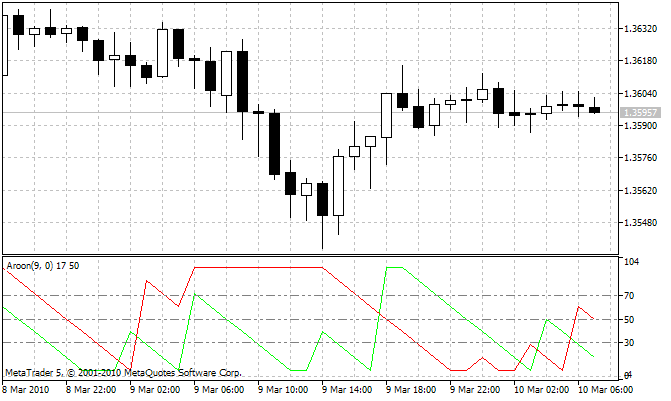To find the position of the element with the maximum or minimum values on a distance no further than AroonPeriod from the current bar we can use built-in ArrayMaximum() and ArrayMinimum() functions of MQL5, which also search for the extremums, but these functions perform the search using the increasing order.

However, the search should be done in decreasing order of indexes. For this case the simplest solution is to change the direction of indexing in the indicator and price buffers, using the ArraySetAsSeries() function.

But we also need to change the direction of the bar ordering in the calculation loop and change the algorithm of the first variable calculation.

In this case the resulting OnCalculate() function will look like this:

```//+------------------------------------------------------------------+
//| Custom indicator iteration function                              |
//+------------------------------------------------------------------+
int OnCalculate(
const int rates_total,    // total number of bars on the current tick
const int prev_calculated,// number of calculated bars on the previous tick
const datetime& time[],
const double& open[],
const double& high[],     // price array for the maximum price for the indicator calculation
const double& low[],      // price array for the minimum price for the indicator calculation
const double& close[],
const long& tick_volume[],
const long& volume[],
)
{
//---+
//--- checking the number of bars
if (rates_total < AroonPeriod - 1)
return(0);

//--- set indexation as timeseries
ArraySetAsSeries(high, true);
ArraySetAsSeries(low,  true);
ArraySetAsSeries(BullsAroonBuffer, true);
ArraySetAsSeries(BearsAroonBuffer, true);

//--- declare the local variables
int limit, bar;
double BULLS, BEARS;

//--- calculation of the starting bar index
if (prev_calculated == 0)                      // check for the first call of OnCalculate function
limit = rates_total - AroonPeriod - 1;  // starting index for the calculation of all of the bars
else limit = rates_total - prev_calculated; // starting index for the calculation of new bars

//--- main loop
for(bar = limit; bar >= 0; bar--)
{
//--- calculation of the indicator values
BULLS = 100 + (bar - ArrayMaximum(high, bar, AroonPeriod) - 0.5) * 100 / AroonPeriod;
BEARS = 100 + (bar - ArrayMinimum(low,  bar, AroonPeriod) - 0.5) * 100 / AroonPeriod;

//--- filling the indicator buffers with the calculated values
BullsAroonBuffer[bar] = BULLS;
BearsAroonBuffer[bar] = BEARS;
}
//----+
return(rates_total);
}
//+------------------------------------------------------------------+```

I changed the name of the variable "first" to "limit", it's more appropriate in this case.

In this case the code of the main loop is similar to way as it was done in MQL4. So, this style of writing the OnCalculate() function can be used for the conversion of indicators from MQL4 to MQL5 with minimal changes of the code.

### Conclusion

So, it's done! The indicator is written, and even in two versions.

For a case of the right, conservative and smart way of solving such problems the solutions turn out slightly more complicated than construction of a some toy using the children's Lego constructor.

Translated from Russian by MetaQuotes Ltd.
Original article: https://www.mql5.com/ru/articles/48

Attached files |
sma_1.mq5 (3.92 KB)
aroon.mq5 (8.04 KB)
aroons.mq5 (6.28 KB)
zigzag.mq5 (9.17 KB)

#### Other articles by this author

Last comments | Go to discussion (1)| 26 May 2023 at 01:13
Once again, Sir, you have blown my mind with a blast from the past. I implemented your MT4-ish loop and its accompanied arrays as series, and created a stellar indicator for MT5. Only one person has posted chart pic of the same indicator that I coded, and that person is smart enough to keep it private. I only wish that foundational material like this could be easier to find in corporately rigged internet searches. 13 years with no prior comments from readers. Unbelievable! Thank you, Nikolay, so much.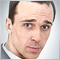Andrey Voitenko: Programming errors cost me \$15,000 (ATC 2010)
Andrey Voitenko is participating in the Automated Trading Championship for the first time, but his Expert Advisor is showing mature trading. For already several weeks Andrey's Expert Advisors has been listed in the top ten and seems to be continuing his positive performance. In this interview Andrey is telling about his EA's features, errors and the price they cost him.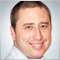Tomasz Tauzowski:"All I can do is pray for a loss position" (ATC 2010)
Tomasz Tauzowski (ttauzo) is a long-standing member of the top ten on the Automated Trading Championship 2010. For the seventh week his Expert Advisor is between the fifth and the seventh places. And no wonder: according to the report of the current Championship leader Boris Odinstov, ttauzo is one of the most stable EAs participating in the competition.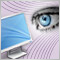Create your own Market Watch using the Standard Library Classes
The new MetaTrader 5 client terminal and the MQL5 Language provides new opportunities for presenting visual information to the trader. In this article, we propose a universal and extensible set of classes, which handles all the work of organizing displaying of the arbitrary text information on the chart. The example of Market Watch indicator is presented.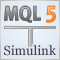Simulink: a Guide for the Developers of Expert Advisors
I am not a professional programmer. And thus, the principle of "going from the simple to the complex" is of primary importance to me when I am working on trading system development. What exactly is simple for me? First of all, it is the visualization of the process of creating the system, and the logic of its work. Also, it is a minimum of handwritten code. In this article, I will attempt to create and test the trading system, based on a Matlab package, and then write an Expert Advisor for MetaTrader 5. The historical data from MetaTrader 5 will be used for the testing process.